# Introduction to theft

#### 2023-10-05

library(dplyr)
library(ggplot2)
library(e1071)
library(theft)

## Purpose

theft facilitates user-friendly access to a structured analytical workflow for the extraction, analysis, and visualisation of time-series features. This structured workflow is presented in the graphic below (note that theft has many more functions than displayed in this graphic—keep reading for more):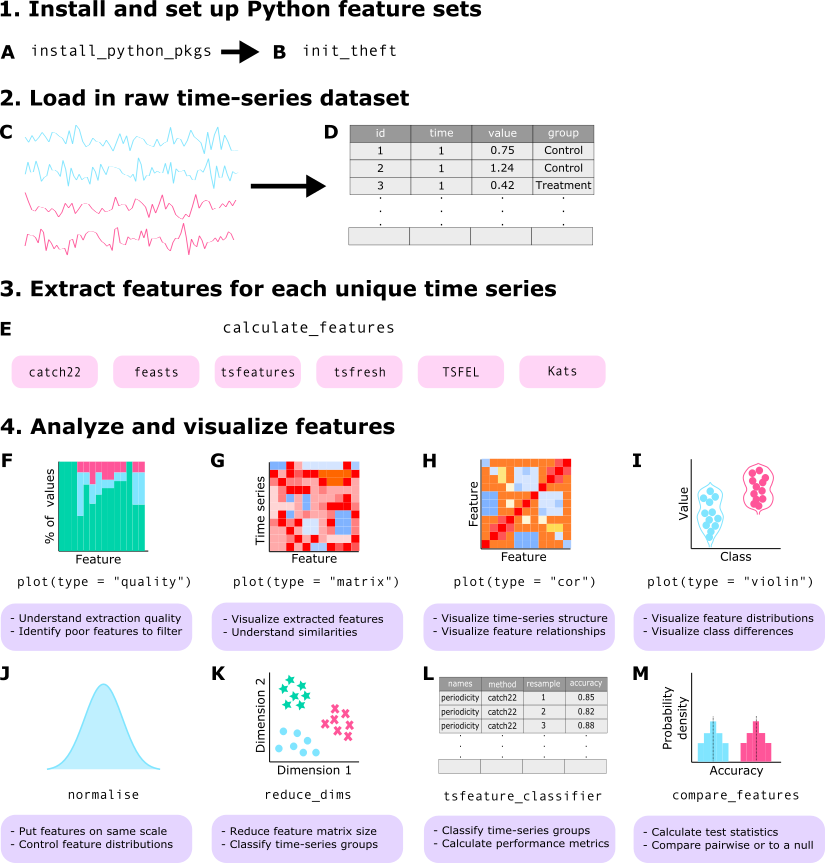## Core calculation functions

To explore package functionality, we are going to use a dataset that comes standard with theft called simData. This dataset contains a collection of randomly generated time series for six different types of processes. The dataset can be accessed via:

theft::simData

The data follows the following structure:

head(simData)
#>                      values timepoint               id        process
#> Gaussian Noise.1 -0.6264538         1 Gaussian Noise_1 Gaussian Noise
#> Gaussian Noise.2  0.1836433         2 Gaussian Noise_1 Gaussian Noise
#> Gaussian Noise.3 -0.8356286         3 Gaussian Noise_1 Gaussian Noise
#> Gaussian Noise.4  1.5952808         4 Gaussian Noise_1 Gaussian Noise
#> Gaussian Noise.5  0.3295078         5 Gaussian Noise_1 Gaussian Noise
#> Gaussian Noise.6 -0.8204684         6 Gaussian Noise_1 Gaussian Noise

### Calculating feature summary statistics

The core function that automates the calculation of the feature statistics at once is calculate_features. You can choose which subset of features to calculate with the feature_set argument. The choices are currently "catch22", "feasts", "Kats", "tsfeatures", "tsfresh", and/or "TSFEL".

Note that Kats, tsfresh and TSFEL are Python packages. The R package reticulate is used to call Python code that uses these packages and applies it within the broader tidy data philosophy embodied by theft. At present, depending on the input time-series, theft provides access to $$>1200$$ features.

#### Installing Python feature sets

Prior to using theft (only if you want to use the Kats, tsfresh or TSFEL feature sets; the R-based sets will run fine) you should have a working Python 3.9 installation and run the function install_python_pkgs(python_path, path) after first installing theft, where the python_path argument is the filepath to the location of Python 3.9 on your machine and the path argument is the location you wish to install the Python libraries and virtual environment to on your machine.

For example, if you wanted to install the Python libraries and the resulting virtual environment in "C:/Users/User/Desktop/theft" and Python 3.9 is located at "/usr/bin/python" on your machine, you would run the following after first having installed theft:

install_python_pkgs("C:/Users/User/Desktop/theft", "/usr/bin/python")

If you want to use any of the Python-based packages, you must first tell R which Python and/or virtual environment on your computer contains the installed libraries. This can be done in theft via the init_theft function, which has two arguments:

1. python_path – the filepath to the version of Python you wish to use (i.e., the same as was entered into install_python_pkgs if you ran that first)
2. venv_path – the filepath to the Python virtual environment where tsfresh, TSFEL, and/or Kats are installed (i.e., the path returned in the console message from install_python_pkgs if you ran that function first)

However, you do not necessarily have to use this convenience function. If you have another method for pointing R to the correct Python (such as reticulate or findpython), you can use those in your workflow instead.

NOTE: You only need to call init_theft or your other solution once per session.

#### Calculating features

You are then ready to use the rest of the package’s functionality, beginning with the extraction of time-series features. Here is an example with the catch22 set:

feature_matrix <- calculate_features(data = simData,
id_var = "id",
time_var = "timepoint",
values_var = "values",
group_var = "process",
feature_set = "catch22",
seed = 123)

Note that for the catch22 set you can set the additional catch24 argument to calculate the mean and standard deviation in addition to the standard 22 features:

feature_matrix <- calculate_features(data = simData,
id_var = "id",
time_var = "timepoint",
values_var = "values",
group_var = "process",
feature_set = "catch22",
catch24 = TRUE,
seed = 123)

A tidy dataframe of most of the included features and the set they correspond to is available in the dataframe feature_list:

head(feature_list)
#>   feature_set                  feature
#> 1     catch22       DN_HistogramMode_5
#> 2     catch22      DN_HistogramMode_10
#> 3     catch22                CO_f1ecac
#> 4     catch22           CO_FirstMin_ac
#> 5     catch22 CO_HistogramAMI_even_2_5
#> 6     catch22            CO_trev_1_num

NOTE: If using the tsfresh feature set, you might want to consider the tsfresh_cleanup argument to calculate_features. This argument defaults to FALSE and specifies whether to use the in-built tsfresh relevant feature filter or not.

### Comparison of feature sets

For a detailed comparison of the six feature sets, see this paper for a detailed review1.

## Data quality checks

The calculate_features function returns an object of class feature_calculations. Objects of this type are purposefully looked-for by other functions in theft. Because it is a class, simple methods such as plot() can be called on the object to produce a range of statistical graphics. The first is a visualisation of the data types of the calculated feature vectors. This is useful for inspecting which features might need to be dropped due to large proportions of undesirable (e.g., NA, NaN etc.) values. We can specify the plot type = "quality to make this graphic:

plot(feature_matrix, type = "quality")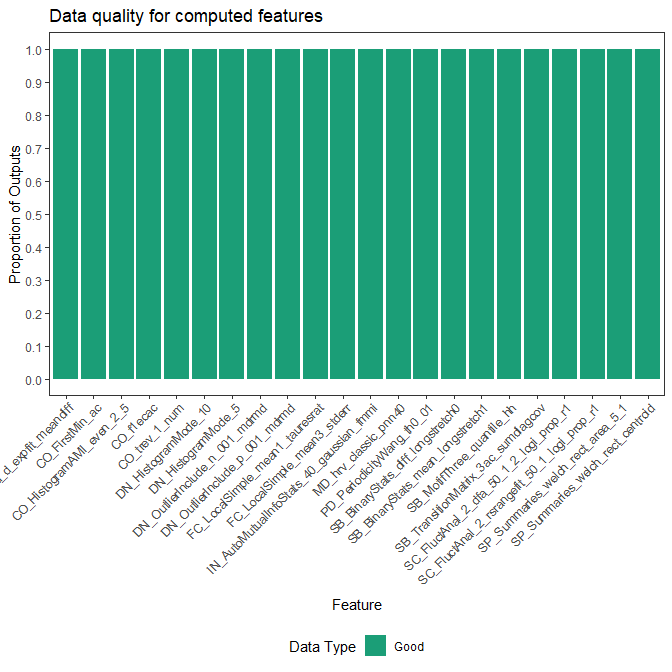## Normalising/scaling functions

Putting calculated feature vectors on an equal scale is crucial for any statistical or machine learning model as variables with high variance can adversely impact the model’s capacity to fit the data appropriately, learn appropriate weight values, or minimise a loss function. theft includes function normalise to rescale either the whole feature_calculations object, or a single vector of values (e.g. values for all participants on just the SB_BinaryStats_mean_longstretch1 feature). Four normalisation methods are offered:

• z-score—"zScore"
• Sigmoid—"Sigmoid"
• Outlier-robust Sigmoid (credit to Ben Fulcher for creating the original MATLAB version) – "RobustSigmoid"
• Min-max—"MinMax"
• Maximum absolute—"MaxAbs"

Normalisation on the whole feature_calculations object can be performed in one line:

normed <- normalise(feature_matrix, norm_method = "zScore", unit_int = FALSE)

For single vector normalisation, all you need to do is pass in a vector as normalise checks for object classes. If you wish to rescale values into the unit interval $$[0,1]$$ after applying the selected normalisation method, you can set unit_int = TRUE.

## Data visualisation and low-dimensional projections

The package also comes with additional statistical and graphical functionality:

• Feature by time-series matrix as a heatmap
• Low dimensional projections of the feature space and plotting as a scatterplot
• Pairwise feature correlation matrix as a heatmap

### Feature matrices

The function calling type = "matrix" in plot() on a feature_calculations object takes itand produces a ggplot object heatmap showing the feature vectors across the x axis and each time series down the y axis. Prior to plotting, the function hierarchically clusters the data across both rows and columns to visually highlight the empirical structure. Note that you have several options for the hierarchical clustering linkage algorithm to use:

• "average" (default)
• "ward.D"
• "ward.D2"
• "single"
• "complete"
• "mcquitty"
• "median"
• "centroid"

See the hclust documentation for more information.

Note that the legend for this plot (and other matrix visualisations in theft) have been discretised for visual clarity as continuous legends can be difficult to interpret meaningful value differences easily.

plot(feature_matrix, type = "matrix", norm_method = "RobustSigmoid")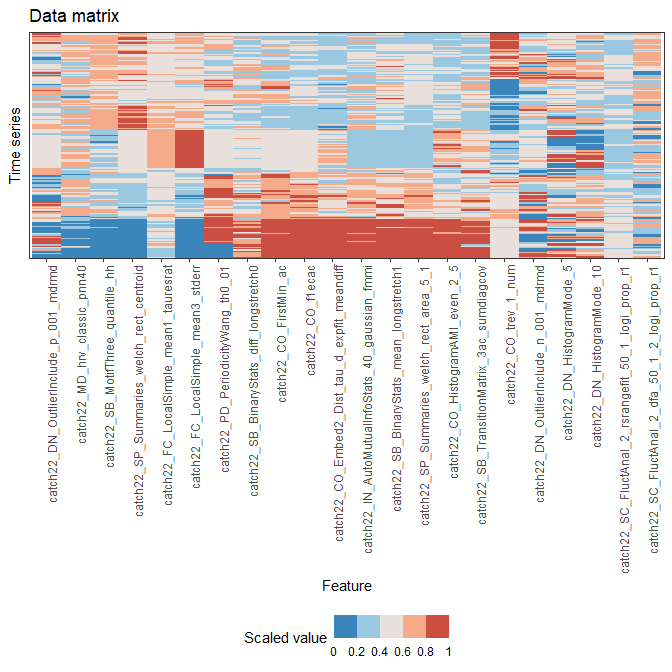You can control the normalisation type with the norm_method argument, whether to rescale to the unit interval after normalisation with the unit_int argument, and the hierarchical clustering method with the clust_method argument (the example above used defaults so manual specification was not needed).

### Individual feature distributions

Plotting the entire feature matrix is useful, but sometimes we wish to understand the distributions of individual features. This is particularly useful if there are different groups in your data (such as in a time-series classification context). We can again use the plot() generic here to draw violin plots through setting type = "violin". Note that for violin plots, we also need to tell the function which features we wish to plot (i.e., a vector of characters specifying feature names from the names column in your feature_calculations object). For simplicity, we will just plot two random features from catch22 here:

plot(feature_matrix, type = "violin",
feature_names = c("CO_f1ecac", "PD_PeriodicityWang_th0_01"))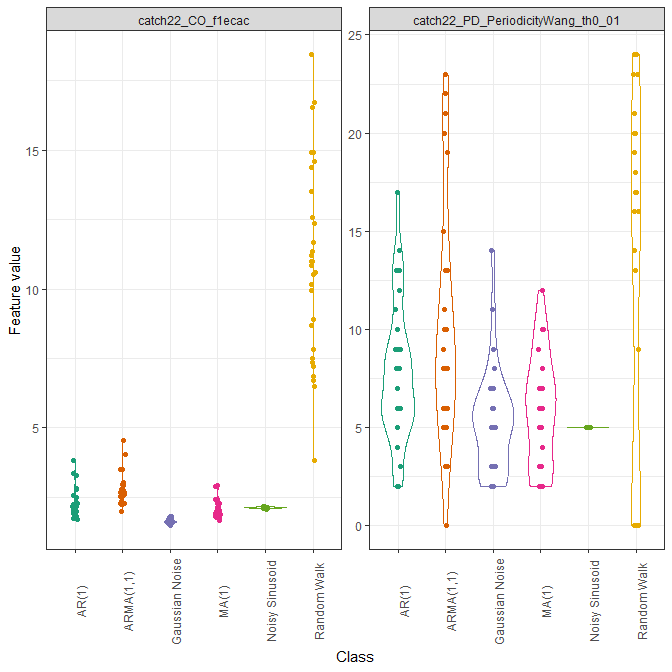Note that when using these defined plot() generics, you can pass any additional arguments to certain geoms to control the plot look through the ... argument in the plot() function. Below is a guide to where these arguments go depending on the plot type:

• type = "quality"... goes to ggplot2::geom_bar
• type = "matrix"... goes to ggplot2::geom_raster
• type = "cor"... goes to ggplot2::geom_raster
• type = "violin"... goes to ggplot2::geom_point

For example, we may wish to control the point size and transparency in the above plot (not rendered here for space):

plot(feature_matrix, type = "violin",
feature_names = c("CO_f1ecac", "PD_PeriodicityWang_th0_01"),
size = 0.7, alpha = 0.9)

### Low dimensional projections

The function reduce_dims takes the feature_calculations object and calculates either a principal components analysis (PCA) or t-distributed stochastic neighbour embedding (t-SNE) fit on it. This result is stored in a custom object class called low_dimension. The function takes the following arguments:

• datafeature_calculations object containing the raw feature matrix produced by calculate_features
• norm_method—character denoting the rescaling/normalising method to apply. Can be one of "zScore", "Sigmoid", "RobustSigmoid", "MinMax", or "MaxAbs". Defaults to "zScore"
• unit_int—Boolean whether to rescale into unit interval $$[0,1]$$ after applying normalisation method. Defaults to FALSE
• low_dim_method—character specifying the low dimensional embedding method to use. Can be one of "PCA" or "tSNE". Defaults to "PCA"
• seed—integer to fix R’s random number generator to ensure reproducibility. Defaults to 123
• ... arguments to be passed to stats::prcomp or Rtsne::Rtsne depending on the selection in low_dim_method
low_dim <- reduce_dims(feature_matrix,
norm_method = "RobustSigmoid",
unit_int = TRUE,
low_dim_method = "PCA",
seed = 123)

We can similarly call plot() on this object to produce a two-dimensional scatterplot of the results:

plot(low_dim)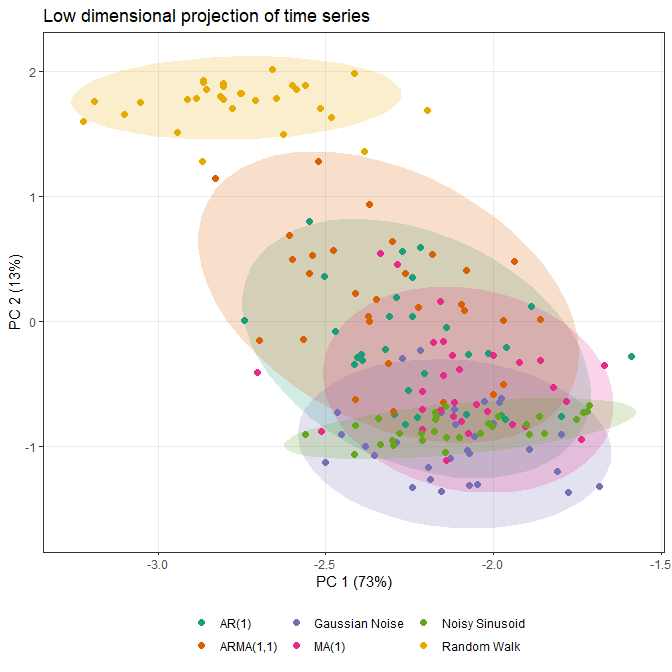Alternatively, a t-SNE version can be specified in a similar fashion, with the perplexity hyperparameter able to be controlled by the user. Typical values for this range between 5 and 50, depending on the size of the data. At lower levels of perplexity, local variations tend to dominate, while at very high levels of perplexity, results can be uninterpretable as clusters can merge. See this interactive article for a detailed review. Shaded covariance ellipses can also be disabled when plotting low_dimension objects by setting show_covariance = FALSE:

low_dim2 <- reduce_dims(feature_matrix,
norm_method = "RobustSigmoid",
unit_int = TRUE,
low_dim_method = "tSNE",
perplexity = 10,
seed = 123)

plot(low_dim2, show_covariance = FALSE)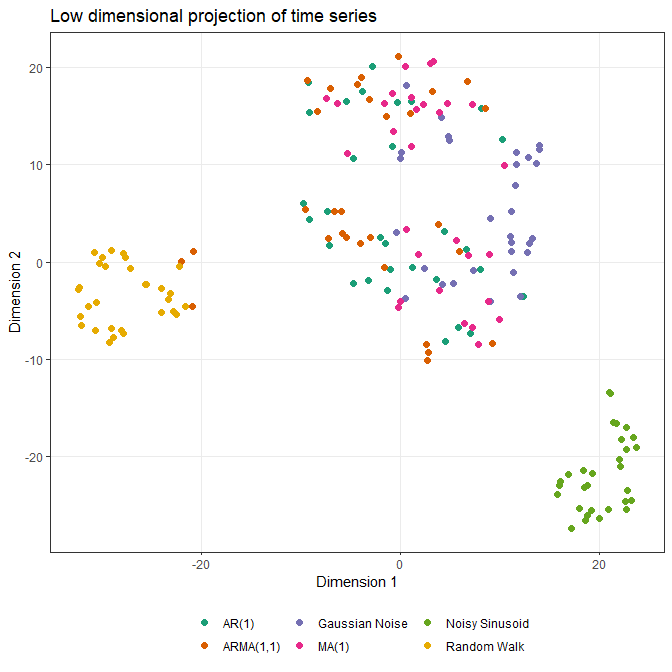There is also the option to specify any other arguments to reduce_dims that are then passed to either stats::prcomp or Rtsne::Rtsne through the ellipsis additional arguments. For example, you may wish to control the maximum number of iterations (max_iter) and the speed-accuracy trade-off (theta) in Rtsne::Rtsne:

low_dim3 <- reduce_dims(feature_matrix,
norm_method = "RobustSigmoid",
unit_int = TRUE,
low_dim_method = "tSNE",
perplexity = 10,
seed = 123,
max_iter = 5000,
theta = 0.2)

You can consult the documentation to get a list of potential arguments by calling either ?prcomp or ?Rtsne.

### Pairwise correlations

You can plot correlations between feature vectors using plot(type = "cor") on a feature_calculations object:

plot(feature_matrix, type = "cor")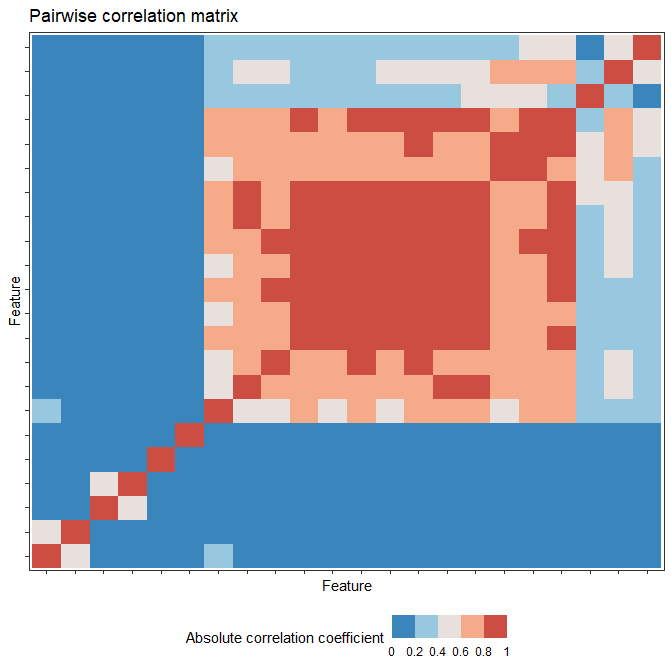Similarly, you can control the normalisation type with the norm_method argument and the hierarchical clustering method with the clust_method argument (the example above used defaults so manual specification was not needed).

## Time-series classification

### Feature-by-feature

Since feature-based time-series analysis has shown particular promise for classification problems, theft includes functionality for exploring group separation. The function tsfeature_classifier enables you to fit a range of classification models to enable statistical comparisons using the resampling methodology presented in this paper for a detailed review2. This function is meant to serve as a fast answer that can be used to guide analysis and not a replacement for the development of a careful statistical pipeline. tsfeature_classifier has the following arguments:

• datafeature_calculations object containing the raw feature matrix produced by calculate_features with an included group column as per theft::calculate_features
• classifierfunction specifying the classifier to fit. Should be a function with 2 arguments: formula and data. Please note that tsfeature_classifier z-scores data prior to modelling using the train set’s information so disabling default scaling if your function uses it is recommended. Defaults to NULL which means the following linear SVM is fit: classifier = function(formula, data){mod <- e1071::svm(formula, data = data, kernel = "linear", scale = FALSE, probability = TRUE)}
• train_size—Numeric value denoting the proportion of samples to use in the training set. Defaults to 0.75
• n_resamples—Integer denoting the number of resamples to calculate. Defaults to 30
• by_set—Boolean specifying whether to compute classifiers for each feature set. Defaults to TRUE (see below section “Multi-feature” for more on this). If FALSE, the function will instead find the best individually-performing features
• use_null—Boolean whether to fit null models where class labels are shuffled in order to generate a null distribution that can be compared to performance on correct class labels. Defaults to FALSE. This is known as permutation testing
• seed—Integer to fix R’s random number generator to ensure reproducibility. Defaults to 123

NOTE: You can filter the duplicate features contained in tsfeatures, feasts, Kats, and catch22 in any feature_calculations object through the function filter_duplicates, which is also called internally in tsfeature_classifier. This function only has 2 arguments: data (the feature_calculations object) and seed. For example, if you assumed that we calculated features from all the sets, you could run the following upfront:

feature_matrix_filt <- filter_duplicates(feature_matrix, seed = 123)

Since we are interested in individual features in this section, we will calculate both main and null results for each feature using just 5 resamples for efficiency (in practice, we would use more!) with the default linear SVM:

feature_classifiers <- tsfeature_classifier(feature_matrix,
by_set = FALSE,
n_resamples = 5,
use_null = TRUE)

To show you how simple it is to specify a different classifier, we can instead maybe use a radial basis function SVM (though you are absolutely not limited to just e1071 models! You can use anything that can be used with R’s predict generic as tsfeature_classifier internally constructs confusion matrices from model predictions):

myclassifier <- function(formula, data){
mod <- e1071::svm(formula, data = data, kernel = "radial", scale = FALSE,
probability = TRUE)
}

classifier = myclassifier,
by_set = FALSE,
n_resamples = 5,
use_null = TRUE)

While have raw classification results is useful, we often also would like to statistical evaluate some facet of it. theft includes the function compare_features for doing this. compare_features contains the following arguments:

• data—List object containing the classification outputs produce by tsfeature_classifier
• metric—Character denoting the classification performance metric to use in statistical testing. Can be one of "accuracy", "precision", "recall", "f1". Defaults to "accuracy"
• by_set—Boolean specifying whether you want to compare feature sets (if TRUE) or individual features (if FALSE). Defaults to TRUE but this is contingent on whether you computed by set or not in tsfeature_classifier
• hypothesis—Character denoting whether p-values should be calculated for each feature set or feature (depending on by_set argument) individually relative to the null if use_null = TRUE in tsfeature_classifier through "null", or whether pairwise comparisons between each set or feature should be conducted on main model fits only through "pairwise". Defaults to "null"
• p_adj—Character denoting the adjustment made to p-values for multiple comparisons. Should be a valid argument to stats::p.adjust. Defaults to "none" for no adjustment. "holm" is recommended as a starting point if adjustments are sought

We can use compare_features to evaluate how well each individual feature performs relative to its empirical null distribution (noting that we are using the defaults for the other arguments for code cleanliness):

feature_vs_null <- compare_features(feature_classifiers,
by_set = FALSE,
hypothesis = "null")

#>                                                 hypothesis
#> 1 catch22_CO_Embed2_Dist_tau_d_expfit_meandiff != own null
#> 2                       catch22_CO_FirstMin_ac != own null
#> 3             catch22_CO_HistogramAMI_even_2_5 != own null
#> 4                            catch22_CO_f1ecac != own null
#> 5                        catch22_CO_trev_1_num != own null
#> 6                  catch22_DN_HistogramMode_10 != own null
#>                                          names
#> 1 catch22_CO_Embed2_Dist_tau_d_expfit_meandiff
#> 2                       catch22_CO_FirstMin_ac
#> 3             catch22_CO_HistogramAMI_even_2_5
#> 4                            catch22_CO_f1ecac
#> 5                        catch22_CO_trev_1_num
#> 6                  catch22_DN_HistogramMode_10
#>                         original_names feature_set   metric feature_mean
#> 1 CO_Embed2_Dist_tau_d_expfit_meandiff     catch22 accuracy   0.32444444
#> 2                       CO_FirstMin_ac     catch22 accuracy   0.30222222
#> 3             CO_HistogramAMI_even_2_5     catch22 accuracy   0.37333333
#> 4                            CO_f1ecac     catch22 accuracy   0.35111111
#> 5                        CO_trev_1_num     catch22 accuracy   0.13333333
#> 6                  DN_HistogramMode_10     catch22 accuracy   0.07555556
#>    null_mean t_statistic     p.value
#> 1 0.04444444   5.5468407 0.002583706
#> 2 0.05333333   5.7154761 0.002317920
#> 3 0.07111111   2.2015813 0.046244746
#> 4 0.06222222   3.5781322 0.011603187
#> 5 0.08000000   1.4446302 0.111034114
#> 6 0.06222222   0.4082483 0.352000000

Or to conduct pairwise comparisons between individual features:

pairwise_features <- compare_features(feature_classifiers,
by_set = FALSE,
hypothesis = "pairwise",

#>                                                                         hypothesis
#> 1           catch22_CO_Embed2_Dist_tau_d_expfit_meandiff != catch22_CO_FirstMin_ac
#> 2 catch22_CO_Embed2_Dist_tau_d_expfit_meandiff != catch22_CO_HistogramAMI_even_2_5
#> 3                catch22_CO_Embed2_Dist_tau_d_expfit_meandiff != catch22_CO_f1ecac
#> 4            catch22_CO_Embed2_Dist_tau_d_expfit_meandiff != catch22_CO_trev_1_num
#> 5      catch22_CO_Embed2_Dist_tau_d_expfit_meandiff != catch22_DN_HistogramMode_10
#> 6       catch22_CO_Embed2_Dist_tau_d_expfit_meandiff != catch22_DN_HistogramMode_5
#>                                        names_a                          names_b
#> 1 catch22_CO_Embed2_Dist_tau_d_expfit_meandiff           catch22_CO_FirstMin_ac
#> 2 catch22_CO_Embed2_Dist_tau_d_expfit_meandiff catch22_CO_HistogramAMI_even_2_5
#> 3 catch22_CO_Embed2_Dist_tau_d_expfit_meandiff                catch22_CO_f1ecac
#> 4 catch22_CO_Embed2_Dist_tau_d_expfit_meandiff            catch22_CO_trev_1_num
#> 5 catch22_CO_Embed2_Dist_tau_d_expfit_meandiff      catch22_DN_HistogramMode_10
#> 6 catch22_CO_Embed2_Dist_tau_d_expfit_meandiff       catch22_DN_HistogramMode_5
#>     metric names_a_mean names_b_mean t_statistic      p.value p_value_adj
#> 1 accuracy    0.3244444   0.30222222   0.3726780 3.641474e-01  1.00000000
#> 2 accuracy    0.3244444   0.37333333  -0.5871366 2.943266e-01  1.00000000
#> 3 accuracy    0.3244444   0.35111111  -1.3093073 1.302873e-01  1.00000000
#> 4 accuracy    0.3244444   0.13333333   4.0273190 7.884261e-03  1.00000000
#> 5 accuracy    0.3244444   0.07555556  22.8619043 1.084307e-05  0.00250475
#> 6 accuracy    0.3244444   0.06222222   7.2623980 9.545688e-04  0.18041351

We can then use ggplot2 to summarise and visualise our results. Here is a pairwise correlation plot between the top 10 features in catch22 for this toy problem. We are just simply filtering the original full feature data and making use of the plot generic defined for objects of class feature_calculations:

top_10 <- feature_vs_null %>%
dplyr::slice_min(p.value, n = 10) %>%
dplyr::select(c(feature_set, original_names, p.value))

feature_matrix_filt <- feature_matrix[] %>%
dplyr::filter(feature_set %in% top_10$feature_set & names %in% top_10$original_names)

feature_matrix_filt <- structure(list(feature_matrix_filt),
class = "feature_calculations")

plot(feature_matrix_filt, type = "cor")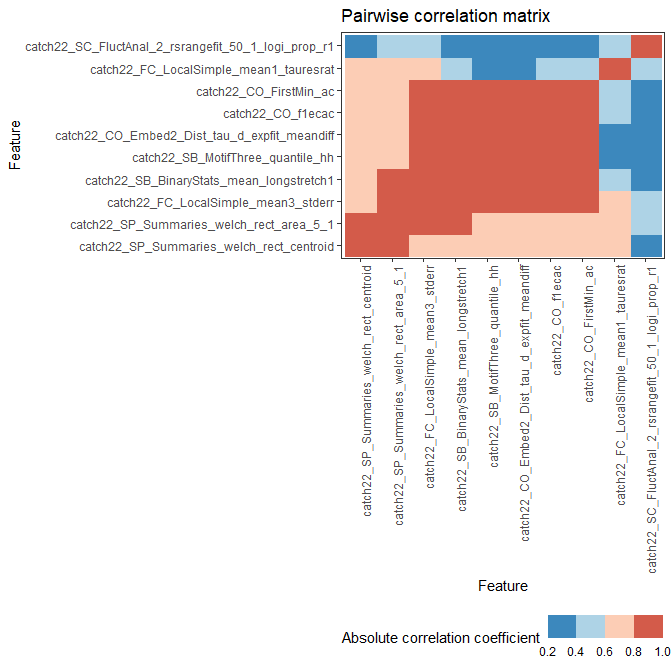We can also easily draw a violin plot of the top 10 features to visualise the distributions by group:

plot(feature_matrix_filt,
type = "violin",
feature_names = top_10$original_names)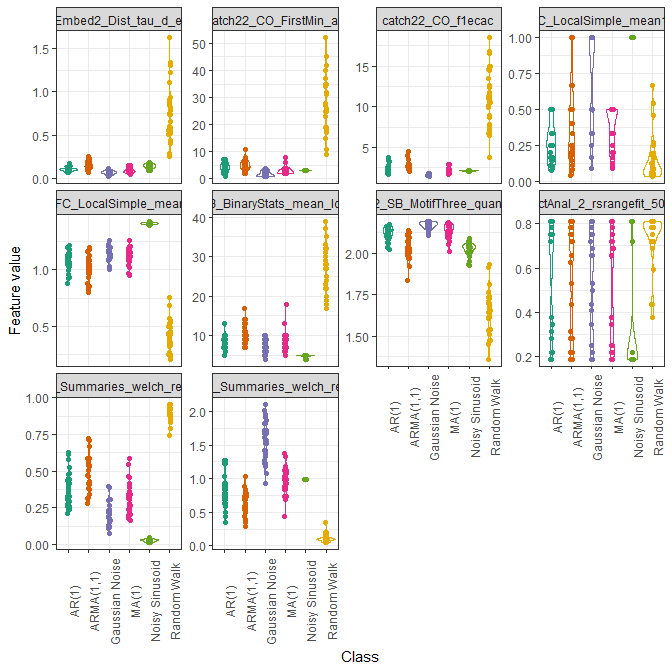Finally, theft also contains a function calculate_interval for summarising the results of tsfeature_classifier. calculate_interval takes the following arguments: • data—list object containing the classification outputs produce by tsfeature_classifier • metric—character denoting the classification performance metric to calculate intervals for. Can be one of "accuracy", "precision", "recall", "f1". Defaults to "accuracy" • by_set—Boolean specifying whether to compute intervals for each feature set. Defaults to TRUE. If FALSE, the function will instead calculate intervals for each feature • type—character denoting whether to calculate a $$\pm$$ SD interval with "sd", confidence interval based off the $$t$$-distribution with "qt", or based on a quantile with "quantile". Defaults to "sd" • interval—numeric scalar denoting the width of the interval to calculate. Defaults to 1 if type = "sd" to produce a $$\pm 1$$ SD interval. Defaults to 0.95 if type = "qt" or type = "quantile" for a $$95\%$$ interval • model_type—character denoting whether to calculate intervals for main models with "main" or null models with "null" if the use_null argument when using tsfeature_classifier was use_null = TRUE. Defaults to "main" We can evidently use calculate_interval to produce a variety of different summaries for us. For example, we might wish to compute the $$\pm1$$ SD interval for each feature’s main model classification accuracy values (note that the defaults for the function do this for us, so we only need to set by_set = FALSE manually): calculate_interval(feature_classifiers, by_set = FALSE) #> # A tibble: 22 × 4 #> names .mean .lower .upper #> <chr> <dbl> <dbl> <dbl> #> 1 catch22_CO_Embed2_Dist_tau_d_expfit_meandiff 0.324 0.299 0.350 #> 2 catch22_CO_FirstMin_ac 0.302 0.262 0.343 #> 3 catch22_CO_HistogramAMI_even_2_5 0.373 0.316 0.431 #> 4 catch22_CO_f1ecac 0.351 0.341 0.361 #> 5 catch22_CO_trev_1_num 0.133 0.0982 0.168 #> 6 catch22_DN_HistogramMode_10 0.0756 0.0502 0.101 #> 7 catch22_DN_HistogramMode_5 0.0622 0.0523 0.0722 #> 8 catch22_DN_OutlierInclude_n_001_mdrmd 0.0667 0.0510 0.0824 #> 9 catch22_DN_OutlierInclude_p_001_mdrmd 0.08 0.0678 0.0922 #> 10 catch22_FC_LocalSimple_mean1_tauresrat 0.387 0.343 0.430 #> # … with 12 more rows ### Multi-feature Since theft contains entire sets of features, we can also use tsfeature_classifier to compare them at the set level through the by_set argument: set_classifiers <- tsfeature_classifier(feature_matrix, by_set = TRUE, n_resamples = 5, use_null = TRUE) head(set_classifiers) #>$TrainTestSizes
#> train_size  test_size
#>        135         45
#>
#> \$ClassificationResults
#>    model_type resample   accuracy mean_precision mean_recall mean_f1_score
#> 1        Main        1 0.73333333      0.7731481   0.7291667     0.7379157
#> 2        Main        2 0.71111111      0.7250000   0.7235450     0.7210240
#> 3        Main        3 0.75555556      0.7643519   0.7500000     0.7481076
#> 4        Main        4 0.71111111      0.7351852   0.7389499     0.7256702
#> 5        Main        5 0.80000000      0.8310185   0.8132937     0.8192783
#> 6        Null        1 0.06666667      0.0915404   0.1083333     0.1173046
#> 7        Null        2 0.08888889      0.0912037   0.0907967     0.1313582
#> 8        Null        3 0.13333333      0.1744108   0.1599327     0.2199786
#> 9        Null        4 0.08888889      0.1100589   0.1079365     0.1306333
#> 10       Null        5 0.28888889      0.2736532   0.2613636     0.3179221
#>    feature_set
#> 1      catch22
#> 2      catch22
#> 3      catch22
#> 4      catch22
#> 5      catch22
#> 6      catch22
#> 7      catch22
#> 8      catch22
#> 9      catch22
#> 10     catch22

Since we only calculated catch22 in this vignette, tsfeature_classifier did not construct a composite set of "All features" (i.e., all features across all computed sets). This set is automatically included by default when $$>2$$ unique feature sets are detected in the feature data. Similar to the individual feature case, we can also use calculate_interval combined with ggplot2 to summarise our findings. Here is a comparison of mean accuracy $$\pm 1SD$$ between feature sets:

interval_calcs <- calculate_interval(set_classifiers)

interval_calcs %>%
ggplot2::ggplot(ggplot2::aes(x = reorder(feature_set, -.mean), y = .mean,
colour = feature_set)) +
ggplot2::geom_errorbar(ggplot2::aes(ymin = .lower, ymax = .upper)) +
ggplot2::geom_point(size = 5) +
ggplot2::labs(x = "Feature set",
y = "Classification accuracy") +
ggplot2::scale_colour_brewer(palette = "Dark2") +
ggplot2::theme_bw() +
ggplot2::theme(legend.position = "none",
panel.grid.minor = ggplot2::element_blank())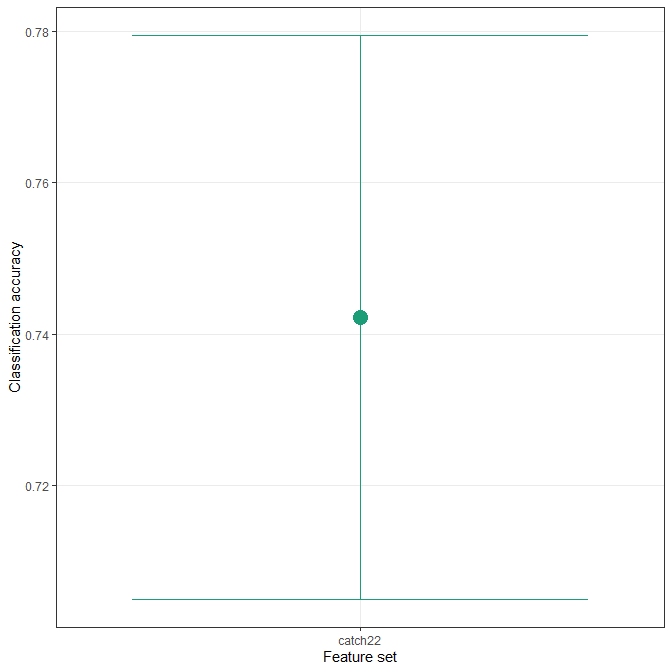## Reading and processing hctsa-formatted files

As theft is based on the foundations laid by hctsa, there is also functionality for reading in hctsa-formatted Matlab files and automatically processing them into tidy dataframes ready for feature extraction in theft. The process_hctsa_file function takes a string filepath to the Matlab file and does all the work for you, returning a dataframe with naming conventions consistent with other theft functionality. As per hctsa specifications for Input File Format 1, this file should have 3 variables with the following exact names: timeSeriesData, labels, and keywords. The filepath can be a local drive path or a URL.

1. T. Henderson and B. D. Fulcher, “An Empirical Evaluation of Time-Series Feature Sets,” 2021 International Conference on Data Mining Workshops (ICDMW), 2021, pp. 1032-1038, doi: 10.1109/ICDMW53433.2021.00134.↩︎

2. T. Henderson, A. G., Bryant, and B. D. Fulcher, “Never a Dull Moment: Distributional Properties as a Baseline for Time-Series Classification”, 27th Pacific-Asia Conference on Knowledge Discovery and Data Mining, 2023.↩︎